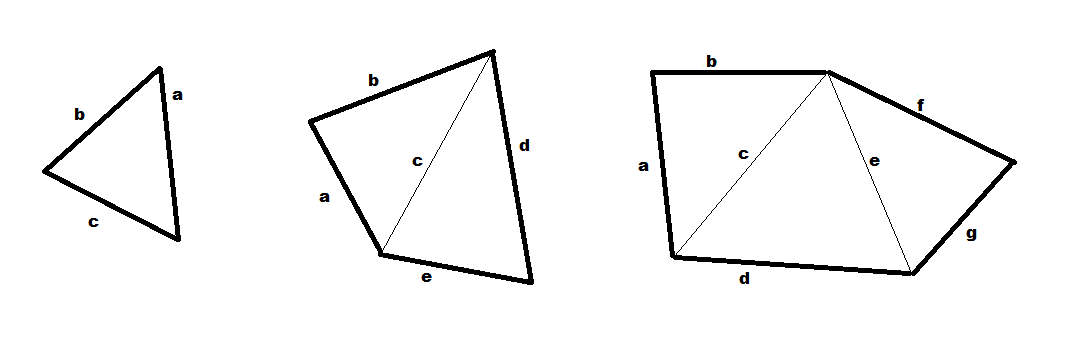# LAND AREA CALCULATOR

### Calculate the area of your land or property

Irregular land area

This tool enables you to calculate the area of your land. What is the surface of your property? Here you can read how to find out the lot size or acreage of your real estate property.

Often a parcel of land is not a rectangle, but it has an odd shape. How to calculate the surface area of irregular shaped fields? You have to split up the land into triangles and for each triangle you need to calculate the area separately. See our image:In order to calculate the area of the triangles, fill in the form below.

First Triangle

Side a:

Side b:

Side c:

Second Triangle

Side c:

Side d:

Side e:

Third Triangle

Side e:

Side f:

Side g: